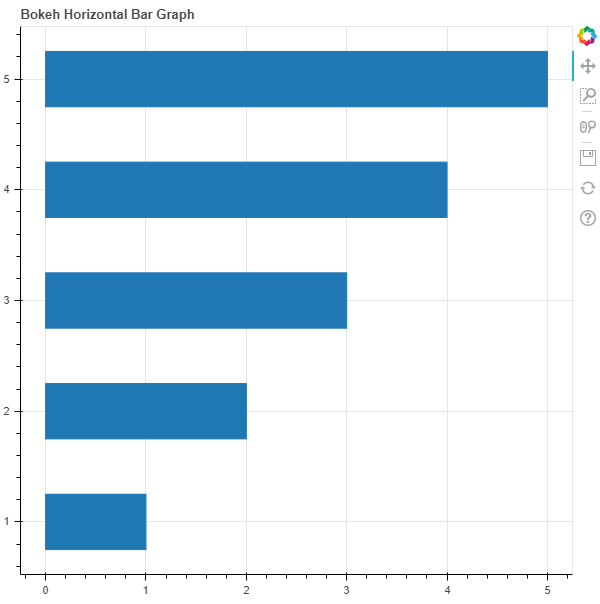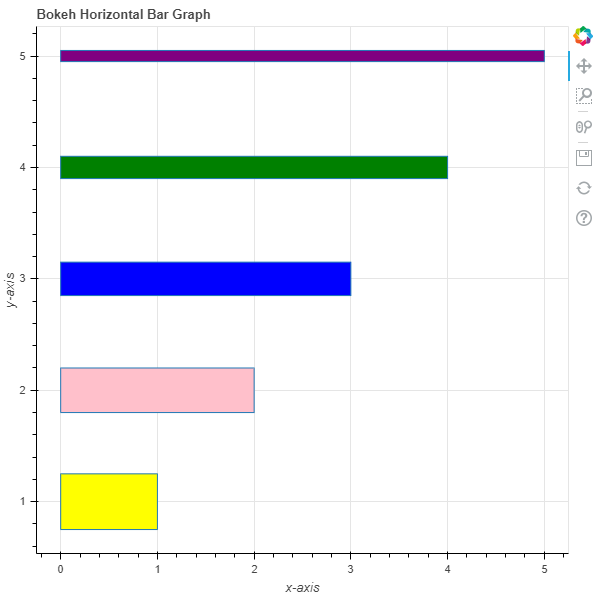# Python Bokeh – Plotting Horizontal Bar Graphs

Bokeh is a Python interactive data visualization. It renders its plots using HTML and JavaScript. It targets modern web browsers for presentation providing elegant, concise construction of novel graphics with high-performance interactivity.

Bokeh can be used to plot horizontal bar graphs. Plotting horizontal bar graphs can be done using the `hbar()` method of the `plotting` module.

## plotting.figure.hbar()

Syntax : hbar(parameters)

Parameters :

• y : y-coordinates of the center of the horizontal bars
• height : thickness of the horizontal bars
• right : x-coordinates of the right edges
• left : x-coordinates of the left edges, default is 0
• fill_alpha : fill alpha value of the horizontal bars
• fill_color : fill color value of the horizontal bars
• hatch_alpha : hatch alpha value of the horizontal bars, default is 1
• hatch_color : hatch color value of the horizontal bars, default is black
• hatch_extra : hatch extra value of the horizontal bars
• hatch_pattern : hatch pattern value of the horizontal bars
• hatch_scale : hatch scale value of the horizontal bars, default is 12
• hatch_weight : hatch weight value of the horizontal bars, default is 1
• line_alpha : percentage value of line alpha, default is 1
• line_cap : value of line cap for the line, default is butt
• line_color : color of the line, default is black
• line_dash : value of line dash such as :
• solid
• dashed
• dotted
• dotdash
• dashdot

default is solid

• line_dash_offset : value of line dash offset, default is 0
• line_join : value of line join, default in bevel
• line_width : value of the width of the line, default is 1
• name : user-supplied name for the model
• tags : user-supplied values for the model

Other Parameters :

• alpha : sets all alpha keyword arguments at once
• color : sets all color keyword arguments at once
• legend_field : name of a column in the data source that should be used
• legend_group : name of a column in the data source that should be used
• legend_label : labels the legend entry
• muted : determines whether the glyph should be rendered as muted or not, default is False
• name : optional user-supplied name to attach to the renderer
• source : user-supplied data source
• view : view for filtering the data source
• visible : determines whether the glyph should be rendered or not, default is True
• x_range_name : name of an extra range to use for mapping x-coordinates
• y_range_name : name of an extra range to use for mapping y-coordinates
• level : specifies the render level order for this glyph

Returns : an object of class `GlyphRenderer`

Example 1 :In this example we will be using the default values for plotting the graph.

 `# importing the modules ` `from` `bokeh.plotting ``import` `figure, output_file, show ` ` `  `# file to save the model ` `output_file(``"gfg.html"``) ` `     `  `# instantiating the figure object ` `graph ``=` `figure(title ``=` `"Bokeh Horizontal Bar Graph"``) ` ` `  `# y-coordinates to be plotted ` `y ``=` `[``1``, ``2``, ``3``, ``4``, ``5``] ` ` `  `# x-coordinates of the right edges ` `right ``=` `[``1``, ``2``, ``3``, ``4``, ``5``] ` ` `  `# height / thickness of the bars  ` `height ``=` `0.5` ` `  `# plotting the graph ` `graph.hbar(y, ` `           ``right ``=` `right, ` `           ``height ``=` `height) ` ` `  `# displaying the model ` `show(graph) `

Output :Example 2 :In this example we will be plotting horizontal bars with different parameters.

 `# importing the modules ` `from` `bokeh.plotting ``import` `figure, output_file, show ` ` `  `# file to save the model ` `output_file(``"gfg.html"``) ` `     `  `# instantiating the figure object ` `graph ``=` `figure(title ``=` `"Bokeh Horizontal Bar Graph"``) ` ` `  `# name of the x-axis ` `graph.xaxis.axis_label ``=` `"x-axis"` `     `  `# name of the y-axis ` `graph.yaxis.axis_label ``=` `"y-axis"` ` `  `# y-coordinates to be plotted ` `y ``=` `[``1``, ``2``, ``3``, ``4``, ``5``] ` ` `  `# x-coordinates of the right edges ` `right ``=` `[``1``, ``2``, ``3``, ``4``, ``5``] ` ` `  `# height / thickness of the bars  ` `height ``=` `[``0.5``, ``0.4``, ``0.3``, ``0.2``, ``0.1``] ` ` `  `# color values of the bars ` `fill_color ``=` `[``"yellow"``, ``"pink"``, ``"blue"``, ``"green"``, ``"purple"``] ` ` `  `# plotting the graph ` `graph.hbar(y, ` `           ``right ``=` `right, ` `           ``height ``=` `height, ` `           ``fill_color ``=` `fill_color) ` ` `  `# displaying the model ` `show(graph) `

Output :Attention geek! Strengthen your foundations with the Python Programming Foundation Course and learn the basics.

To begin with, your interview preparations Enhance your Data Structures concepts with the Python DS Course.

My Personal Notes arrow_drop_upCheck out this Author's contributed articles.

If you like GeeksforGeeks and would like to contribute, you can also write an article using contribute.geeksforgeeks.org or mail your article to contribute@geeksforgeeks.org. See your article appearing on the GeeksforGeeks main page and help other Geeks.

Please Improve this article if you find anything incorrect by clicking on the "Improve Article" button below.

Article Tags :

Be the First to upvote.

Please write to us at contribute@geeksforgeeks.org to report any issue with the above content.